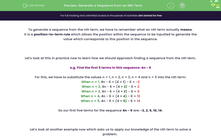# Generate a Sequence from an Nth Term

In this worksheet, students use nth terms to find specified values in a sequence and to solve problems involving the relationships between terms in a sequence.Key stage:  KS 4

Year:  GCSE

GCSE Subjects:   Maths

GCSE Boards:   AQA, Eduqas, Pearson Edexcel, OCR,

Curriculum topic:   Algebra

Curriculum subtopic:   Sequences

Difficulty level:#### Worksheet Overview

To generate a sequence from the nth term, we have to remember what an nth term actually means.

It is a position-to-term rule which allows the position within the sequence to be inputted to generate the value which corresponds to this position in the sequence.

Let's look at this in practice now to learn how we should approach finding a sequence from the nth term.

e.g. Find the first 5 terms in this sequence: 4n - 6

For this, we have to substitute the values n = 1, n = 2, n = 3, n = 4 and n = 5 into the nth term:

When n = 1, 4n - 6 = (4 × 1) - 6 = -2

When n = 2, 4n - 6 = (4 × 2) - 6 = 2

When n = 3, 4n - 6 = (4 × 3) - 6 = 6

When n = 4, 4n - 6 = (4 × 4) - 6 = 10

When n = 5, 4n - 6 = (4 × 5) - 6 = 14

So our first five terms for the sequence 4n - 6 are: -2, 2, 6, 10, 14.

Let's look at another example now which asks us to apply our knowledge of the nth term to solve a problem.

e.g. Find the difference between the 100th and the 90th terms in this sequence: 5n + 2

The approach to this is very similar to the last question, but we use n = 90 and n = 100 instead:

When n = 100, 5n + 2 = (5 × 100) +2 = 502

When n = 90, 5n + 2 = (5 × 90) +2 = 452

We can then find the difference between these two terms by subtracting these two values:

502 - 450 = 50

So the difference between the 90th and 100th terms in the sequence 5n + 2 is 50

In this activity, we will use nth terms to find specified values in a sequence and to solve problems involving the relationships between terms in a sequence.

### What is EdPlace?

We're your National Curriculum aligned online education content provider helping each child succeed in English, maths and science from year 1 to GCSE. With an EdPlace account you’ll be able to track and measure progress, helping each child achieve their best. We build confidence and attainment by personalising each child’s learning at a level that suits them.

Get started